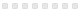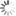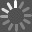# Just-in-time

## Algebra and Trigonometry for Early Transcendental Calculus

Book - 2006
Rate this:PEARSON

Strong algebra and trigonometry skills are crucial to success in calculus. This text is designed to bolster these skills while readers study calculus. As readers make their way through the calculus course, this supplemental text shows them the relevant algebra or trigonometry topics and points out potential problem spots. The table of contents is organized so that the algebra and trigonometry topics are arranged in the order in which they are needed for calculus.

Numbers and Their Disguises: Multiplying and dividing fractions, adding and subtracting fractions, parentheses, exponents, roots, percent, scientific notation, calculators, rounding, intervals. Completing the Square: Completing the square in one and two variables. Solving Equations: Equations of degree 1 and 2, solving other types of equations, rational equations, the zero-factor property. Functions and Their Graphs: Introduction, equations of lines, power functions, shifting graphs, intersection of curves. Cyclic Phenomena: The Six Basic Trigonometric Functions: Angles, definitions of the six trigonometric functions, basic identities, special angles, sum formulas. Exponential Functions: The family of exponentials, the function. Composition and Inverse Functions: Composite functions, the idea of inverses, finding an inverse of f given by a graph, finding the inverse of f given by an expression. Logarithmic Functions: Definition of logarithms, logs as inverses of exponential functions, laws of logarithms, the natural logarithm. Inverse Trigonometric Functions: The definition of arcsin x, the functions arctan x and arcsec x, inverse trigonometric identities. Changing the Form of a Function: Factoring, canceling, long division, rationalizing, extracting a factor from under a root. Simplifying Algebraic Expressions: Working with difference quotients and rational functions, canceling common factors, rationalizing expressions. Decomposition of Functions: Inner, outer, and outermost functions, decomposing composite functions. Equations of Degree 1 Revisited: Solving linear equations involving derivatives. Word Problems, Algebraic and Transcendental: Algebraic word problems, the geometry of rectangles, circles and spheres, trigonometric word problems, right angle triangles, the law of sines and the law of cosines, exponential growth and decay. Trigonometric Identities: Rewriting trigonometric expressions using identities.

For all readers interested in algebra and trigonometry in early transcendentals calculus.

Publisher: Boston : Pearson Addison-Wesley, c2006
Edition: 3rd ed
ISBN: 9780321320506
0321320506
Characteristics: ix, 214 p. ; 26 cm## Opinion

### From the critics### Community Activity

#### Comment

There are no comments for this title yet.

#### Age

There are no ages for this title yet.

#### Summary

There are no summaries for this title yet.

#### Notices

There are no notices for this title yet.

#### Quotes

There are no quotes for this title yet.## Explore FurtherLoading...Loading...